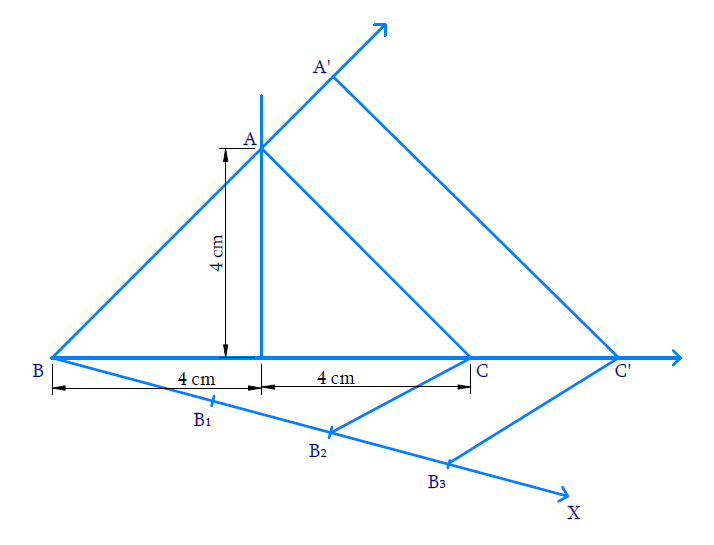# Ex.11.1 Q4 Constructions Solution - NCERT Maths Class 10

Go back to  'Ex.11.1'

## Question

Construct an isosceles triangle whose base is $$8 \;\rm{cm}$$ and altitude $$4 \;\rm{cm}$$ and then another triangle whose sides are \begin{align}1\frac{1}{2} \end{align} times the corresponding sides of the isosceles triangle.

Video Solution
Constructions
Ex 11.1 | Question 4

## Text Solution

#### What is known?

Base and altitude of an isosceles triangle and the ratio of corresponding sides of $$2$$ triangles.

Construction.

#### Reasoning:

• Draw the line segment of base $$8 \;\rm{cm}$$. Draw perpendicular bisector of the line. Mark a point on the bisector which measures $$4 \;\rm{cm}$$ from the base. Connect this point from both the ends.
• Then draw another line which makes an acute angle with the given line. Divide the line into $$m + n$$ parts where $$m$$ and $$n$$ are the ratio given.
• Two triangles are said to be similar if their corresponding angles are equal. They are said to satisfy Angle-Angle-Angle (AAA) Axiom.
• Basic proportionality theorem states that, “If a straight line is drawn parallel to a side of a triangle, then it divides the other two sides proportionally".

#### Steps:Steps of construction:

(i) Draw $$\,{{BC = 8}}\,{\rm{cm}}{{.}}$$ Through $$D,$$ the mid-point of $$BC,$$ draw the perpendicular to $$BC$$ and cut an arc from $$D$$ on it such that $${{DA = 4}}\,{{cm}}{{.}}$$ Join $$BA$$ and $$CA.$$ $${{\Delta ABC}}$$ is obtained.

(ii) Draw the ray $$BX$$ so that $$\angle {{CBX}}$$ is acute.

(iii) Mark $$3$$ \begin{align}\left( {3{{ }} > {{ }}\;2\text{ in}\,\,1\frac{1}{2} = \,\frac{3}{2}} \right)\end{align}  points $${{{B}}_{{I}}}{{,}}\,{{{B}}_{{2}}}{{,}}\,{{{B}}_{{3}}}$$ on BX such that $${{B}}{{{B}}_{{1}}}{{ = }}{{{B}}_{{1}}}{{{B}}_{{2}}}{{ = }}{{{B}}_{{2}}}{{{B}}_{{3}}}$$

(iv) Join $${B}_{2}$$ ($$\;2^\rm{nd}$$  point $$∵ \;2<3$$) to C and draw $${B}_{3} {C}^{\prime}$$ parallel to $${B}_{2} {C}$$ intersect $$BC$$ extended at $$C’$$.

(v)  Through $$C’$$ draw $${{C'A'}}$$ parallel to $$CA$$ to intersect $$BA$$ extended to $$A’.$$

Now, $${{\Delta A'BC'}}$$ is the required triangle similar to $$\,{{\Delta ABC}}$$ where

\begin{align}\frac{{{{BA'}}}}{{{{BA}}}}{{ = }}\frac{{{{C'A'}}}}{{{{CA}}}}{{ = }}\frac{{{{BC'}}}}{{{{BC}}}}{{ = }}\frac{{{3}}}{{{2}}}\end{align}

Proof:

In $${{\Delta B}}{{{B}}_{{3}}}{{C',}}\,\,{{{B}}_{{2}}}{{C}}\,\,{{||}}\,\,{{{B}}_{{3}}}{{C'}}$$ ,

Hence by Basic proportionality theorem,

\begin{align}\frac{{{{{B}}_{{2}}}{{{B}}_{{3}}}}}{{{{B}}{{{B}}_{{2}}}}}{{ = }}\frac{{{{CC'}}}}{{{{BC}}}}{{ = }}\frac{{{1}}}{{{2}}}\end{align}

Adding $$1$$,

\begin{align}\frac{{{{CC'}}}}{{{{BC}}}}{{ + 1}}&= \frac{{{1}}}{{{2}}}{{ + 1}}\\\frac{{{{BC + CC'}}}}{{{{BC}}}}{{ }}&=\frac{{{3}}}{{{2}}}\\\frac{{{{BC'}}}}{{{{BC}}}}{{}}&= \frac{{{3}}}{{{2}}}\end{align}

Consider $$\,{{\Delta BAC }}\,{{and }}\,{{\Delta BA'C'}}$$

$$\angle {{ABC}}\, = \,\angle {{A'BC'}}$$ (Common)

$$\angle {BCA}=\angle {BC' } { A '}$$ (Corresponding angles $$\therefore \mathrm{CA} \| \mathrm{C}^{\prime} \mathrm{A}^{\prime}$$

$$\angle {{BAC}}\, = \,\angle {{BA'C'}}$$ (Corresponding angles)

By AAA axiom, $${{\Delta BAC \sim\Delta BA'C'}}$$

$$∴$$ Corresponding sides are proportional

Hence,

\begin{align}\frac{{{{BA'}}}}{{{{BA}}}}{{ = }}\frac{{{{BC'}}}}{{{{BC}}}}{{ = }}\frac{{{{CA'}}}}{{{{CA}}}}{{ = }}\frac{{{3}}}{{{2}}}\end{align}

Learn from the best math teachers and top your exams

• Live one on one classroom and doubt clearing
• Practice worksheets in and after class for conceptual clarity
• Personalized curriculum to keep up with school